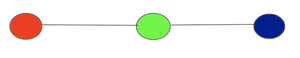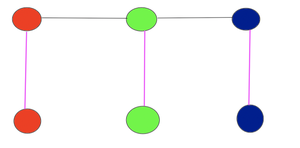# Prove that KITE is NP-Complete

• Last Updated : 22 Aug, 2022

Prerequisite: NP-Completeness, NP Class, Clique

Problem: Given an undirected graph G = (V, E) and an integer K, determine if there is a kite subgraph of size 2K which consists of a clique of size K with a K-size tail. Demonstrate that it is an NP-Complete.

Explanation:

A KITE is a graph on an even number of vertices, say 2k, in which k of the vertices form a clique and the remaining K vertices are connected in a “tail” that consists of a path joined to one of the vertices of the clique.

Given KITE problem can be described as follows:

• Input –  Graph G (V, E) having an even number of vertices (say 2K).
• Output –  K of the vertices form a clique and remaining K vertices are connected in a “tail” that consists of a path joined to one of the
vertices of the clique.

To prove a problem NP Complete , there are two steps involved:

1. Prove given problem belong to NP Class
2. All other problems in the NP class can be polynomial time reducible to that problem. (This is the prove of being NP-Hard)

Now it is not possible to reduce every NP problem to another NP problem to prove it’s NP completeness all the time. That’s why we show that any known NP complete problem is reducible to that problem in polynomial time.

Proof:

1. KITE belongs to NP Class:

A problem is said to be in NP Class if the solution for the problem can be verified in polynomial time.

Given an input G = (V, E) and a set size of K, the answer is K of the vertices form a clique and the remaining K vertices are connected in a “tail” that consists of a path joined to one of the
vertices of the clique. There are two steps to verify the solution for this problem:

• Verify K of the vertices form a clique: For all pairs of vertices (x, y) such that (x, y) ∈ E. This would take O(n2) time  K ≤ n where n is the number of vertices.
• Verify remaining K vertices are connected in a “tail” that consists of a path joined to one of the vertices of the clique. This would take O(n2) time  K ≤ n where n is the number of vertices.

So, verification of a solution for KITE at most O(n2) which is polynomial in nature so KITE belongs to NP Class.

2. KITE is an NP-Hard problem:

Now we need to show KIte is at least as hard as a known NP-Complete Problem by reduction technique.

Here the known problem is going to be the Clique problem which is already known to be NP-complete which is explained in Proof of Clique being the NP-Complete.

We are going to show the reduction from Clique -> KITE.

Input Conversion: We need to convert the input from Clique to input to KITE

Clique takes an input graph G = (V, E) and parameter K for set size. To convert the input from Clique to KITE:Clique graph G = (V,E) with 3 vertices and 2 edges

• We will create a graph such as  G’(V’, E’) which is going to be the copy of the given graph G = (V, E)
• We are going to add |V| the vertices from G to G’ such that V’ = 2|V|.
• Now add an edge between newly added vertices with its original vertex in G’.Kite graph G’= (V’, E’), where all newly added vertices are connected with its original vertices using pink line

So, Graph G’ is going to be the graph that will have an even number of vertices, say K = 2V, in which V of the vertices form a clique and the remaining V vertices are connected in a “tail” that consists of a path joined to one of the vertices of the clique.

The creation of a new graph is going to take O(|n| + |m|) time. Adding new vertices is going to take O(n),  where n is the number of vertices and m are the edges. Thus the input conversion can be done in polynomial time.

Output Conversion: We need to convert the solution from KITE to the solution for the Clique problem.

• KITE solution yields 2 sets of vertices where V of the vertices form a clique and the remaining V vertices are connected in a “tail” that consists of a path joined to one of the vertices of the clique.
• This solution can be transformed into a Clique problem solution by removing the edges between the vertices which are connected in a tail from graph G'(V’, E’), resulting in Graph G(V, E), with Clique for G equal to Clique for G‘ because there is no edge difference between G and G’.

The vertices can be dropped in O(n) where n is the number of vertices, thus the output conversion can be done in polynomial time.

Correctness: Now we need to prove the correctness of the claim which says that

“Solution of KITE exits iff a solution to the Clique problem exists”.

• Forward implication: For a kite graph having two different sets of vertices where V of the vertices form a clique and the remaining V vertices are connected in a “tail” that consists of a path joined to one of the vertices of the clique. We can drop the vertices connected with thetail and the resulting graph would be a clique.
• Reverse implication:  For a graph G= (V, E) having a clique, then a kite graph also exists by adding all the vertices |V| and connecting them with a tail which will also form a clique

So, this means KITE Graph has a solution ↔  Clique Graph has a solution.

Conclusion:

Hence, we can conclude that KITE is an NP-complete problem.

My Personal Notes arrow_drop_up